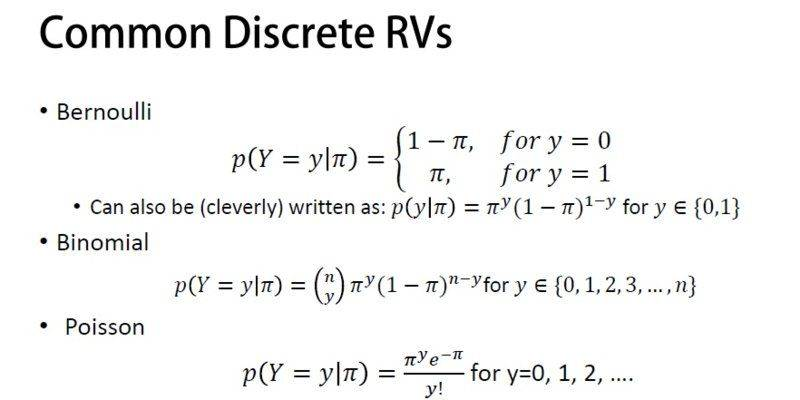# Bernoulli, Binomial & Poisson: What is pi?

• zak100

## Homework Statement

Hi,
I have a confusion in knowing Pi in the equations attached. Eq are related to the Topic Discrete Random Variables in the context of Probability lecture
I also can't understand what is P(Y=y|Pi)?

Eq are attached

## The Attempt at a Solution

I can't understand the meaning of Pi. Does it mean probability of success?

Zulfi.## Homework Statement

Hi,
I have a confusion in knowing Pi in the equations attached. Eq are related to the Topic Discrete Random Variables in the context of Probability lecture
I also can't understand what is P(Y=y|Pi)?

Eq are attached

## The Attempt at a Solution

I can't understand the meaning of Pi. Does it mean probability of success?

Zulfi.View attachment 230451
It looks like ##\pi## just represents the parameter of the distribution. So this is not the common definition ##\pi = 3.14##... In the Bernoulli and Binomial cases, ##\pi## is the probability of success, and in the Poisson example, ##\pi## is the average rate of occurrence.

•zak100
Here pi is the "success" probability for the event being counted in each distribution. Bernoulli is basically Binomial with n=1.

•zak100

## Homework Statement

Hi,
I have a confusion in knowing Pi in the equations attached. Eq are related to the Topic Discrete Random Variables in the context of Probability lecture
I also can't understand what is P(Y=y|Pi)?

Eq are attached

## The Attempt at a Solution

I can't understand the meaning of Pi. Does it mean probability of success?

Zulfi.View attachment 230451

Typically, for bernoulli/binomial distributions we have a single-trial "success" probability, often denoted as ##p## but sometimes as ##\pi## or some other symbol. Note that the parameter is in the range from 0 to 1, because it is a probability.

For Poisson distributions we are typically counting something (like the number of successes or failures, or the number of customers arriving at a store, or the number of radioactive decays, etc.) The mean and variance depend on some parameter, and your book is calling that parameter ##\pi##. However, unlike the bernoulli/binomial case, ##\pi## can be any positive number and need not be less than 1.

•zak100
Hi,
What is meant by P(Y=y|Pi) in the above equations?

Zulfi.

Hi,
•